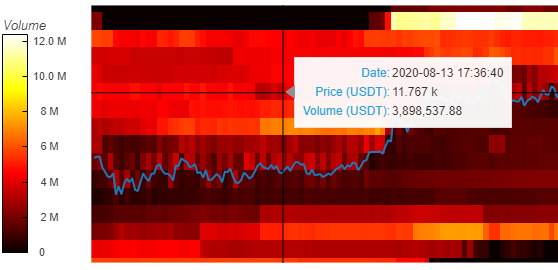# Hvplot heatmap grouby opts change default tooltips

1. I have a data frame in following format

1. Now i want to change the tooltips in MBU_heat with following code

from bokeh.models import HoverTool
from holoviews import opts

hover=HoverTool(tooltips=[(‘Date’, ‘@{ddate}{%F}’),(‘MBU’,’@{MBU#}’),(‘Count’,’@{Count}’)], formatters={’@{ddate}’: ‘datetime’})

opts.defaults(opts.HeatMap(toolbar=‘above’, tools=[‘hover’]))

1. Now i create a its heatmap with hvplot and groupby options with following code and output

`MBU_heat=mbu_comp.hvplot.heatmap( title='MBU',x='ddate', y='MBU#', C='Count' ,reduce_function=np.sum ,groupby='RBU', widget_location='top_left',cmap=LSM_cmap,colorbar=True,height=400,width=700)`

1. Following are the status of MBU_heat

1. tooltip does not apply on the heatmap .

2. if I use via %opts or %% in notebook it works fine but i want to use in python program

3. Kindly guide how i apply and access opts tools tip in MBU_heat objects in python program

4. or how if can use %opts or %%opts in python program

I shall be very thankful

formating of the date not working in tooltip

Have you tried:

`MBU_heat=mbu_comp.hvplot.heatmap( title='MBU',x='ddate', y='MBU#', C='Count' ,reduce_function=np.sum ,groupby='RBU', widget_location='top_left',cmap=LSM_cmap,colorbar=True,height=400,width=700).opts(tools=[hover])`

?

Regarding the formatting. Sometimes hvplot screws up the variables, so maybe it got re-assigned to ‘x’:

For me, hvplot of an xarray object resulted in me having to use the following tooltip, since my dimension names got reassigned:

``````tooltips = [
('Date', '\$x'),
(f'Price ({quote_currency})', '\$y{'+f'{formatting}0'+' a}'),
(f'Volume ({quote_currency})', '@image{0,0.00}')
]
hover = HoverTool(tooltips=tooltips)
``````BTW, a useful way to find out your current plot’s hover variables is this:

``````renderer = hv.renderer('bokeh')
renderer.get_plot(your_plot_object).handles['hover_tools']``````

Deleting the quotes around hover in this line should do the trick:
opts.defaults(opts.HeatMap(toolbar=‘above’, tools=[‘hover’]))

1 Like

this works. Thanks a lot

1 Like

It results to following error

AttributeError: ‘Column’ object has no attribute ‘opts’

print(MBU_heat)

Column
 Row
 WidgetBox
 Select(margin=(20, 20, 20, 20), name=‘RBU’, options=[‘Central A’, …], value=‘Central A’, width=250)
 HSpacer()
 HoloViews(DynamicMap, widget_location=‘top_left’)